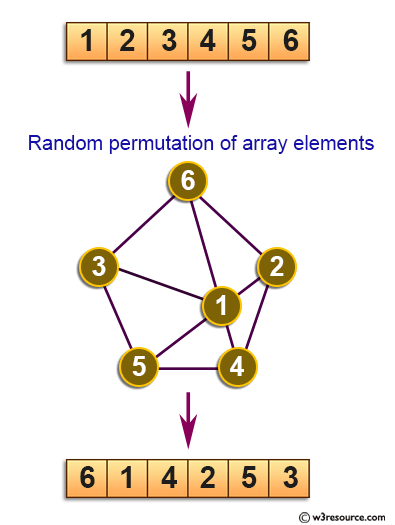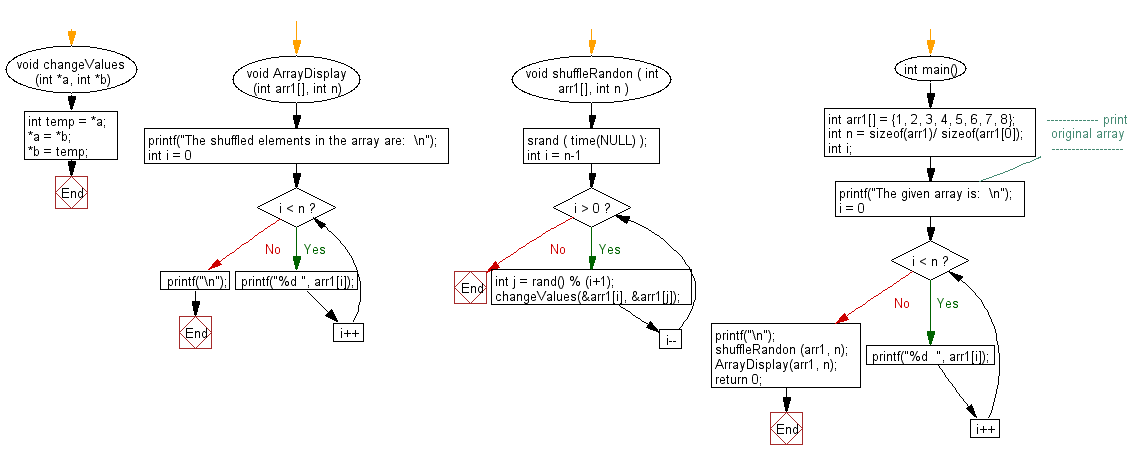﻿ C exercises: Generate a random permutation of array elements - w3resource# C Exercises: Generate a random permutation of array elements

## C Array: Exercise-77 with Solution

Write a program in C to generate a random permutation of array elements.

Pictorial Presentation:Sample Solution:

C Code:

``````#include <stdio.h>
#include <stdlib.h>
#include <time.h>

void changeValues (int *a, int *b)
{
int temp = *a;
*a = *b;
*b = temp;
}

void ArrayDisplay (int arr1[], int n)
{
printf("The shuffled elements in the array are:  \n");
for (int i = 0; i < n; i++)
printf("%d ", arr1[i]);
printf("\n");
}
void shuffleRandon ( int arr1[], int n )
{
srand ( time(NULL) );
for (int i = n-1; i > 0; i--)
{
int j = rand() % (i+1);
changeValues(&arr1[i], &arr1[j]);
}
}
int main()
{
int arr1[] = {1, 2, 3, 4, 5, 6, 7, 8};
int n = sizeof(arr1)/ sizeof(arr1);
int i;
//------------- print original array ------------------
printf("The given array is:  \n");
for(i = 0; i < n; i++)
{
printf("%d  ", arr1[i]);
}
printf("\n");
//------------------------------------------------------
shuffleRandon (arr1, n);
ArrayDisplay(arr1, n);
return 0;
}
```
```

Sample Output:

```The given array is:
1  2  3  4  5  6  7  8
The shuffled elements in the array are:
2 8 7 3 4 5 1 6
```

Flowchart:C Programming Code Editor:

Improve this sample solution and post your code through Disqus.

What is the difficulty level of this exercise?

﻿